# Mathematical Software for Windows

Among our mathematical software for Windows you'll be able to find applications to carry out all sorts of calculations and graphical representations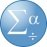IBM SPSS Statistics 28.0.1.0 (142)

Poweful software to collect and analyze statistical data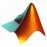MATLAB R2022a

Maths software tool for PC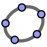GeoGebra 6.0.729

Software to learn maths at all levels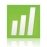Minitab 19.1.1.0

One of the best statistical calculation programs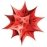Mathematica 12.0.0

Platform for computational development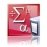PASW Statistics 18.0.0

One of the most powerful statistic solutions on the market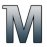Software for engineering calculations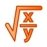Solve mathematical operations with this free tool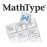MathType 7.3.1.438

Complete mathematical equation and formula editor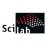Scilab 6.0.2

The most comprehensive tool to perform numerical calculations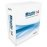Solve complex mathematical operations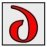Powerful tool for symbolic mathematic calculations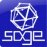SageMath 8.9 v0.5.1

Open-source alternative to Matlab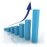Statgraphics 18.1.08

Carry out statistical analyses on your data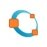Octave 4.4.1

Applied calculus software very similar to MATLAB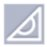GeoEnZo 4.7.0.0

Digital board to teach and learn maths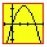WinPlot 1.55

Generate 2D and 3D graphics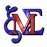Maxima 5.44.0

Excellent free computer algebra system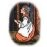Gretl 2021d

Application for econometric analyses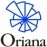Oriana 4.02

Tool to view and analyze circular data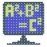Mathomatic 16.0.5

Light software for the calculation of computational algebra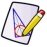Geometry 2.8.2

Complete trigonometry and geometry calculations easily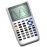Maths tool to solve equations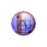Observe and measure your diagrams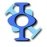FreeMat 4.2

Free numerical calculation environment that is an alternative to MATLAB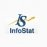Infostat 2018d

Software for statistical analysis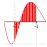Calculate and view maths charts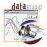DataStudio 1.9.8.10

View and analyze the data of your experiments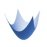Complete numerical and algebraic computation system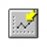FindGraph 2.611

Powerful program to generate tables and 2D graphs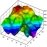DPlot 2.3.5.7

Generate all kinds of 2D and 3D charts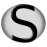SMath Studio 0.99.6884

Develop maths operations in this virtual notebook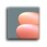Zhu3D 4.2.2

View any mathematical function in 3D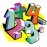Rlab 2.1.05b

Develop your own maths applications with this quick program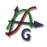Graph 4.4.2.543

Excellent application to draw maths graphs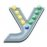Yacas 1.6.1

A computer algebra system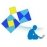Geometer 1.7.0

An application to calculate surfaces and volumes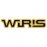Application to carry out calculations and graphic representations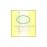Excellent tool to create all sorts of maths graphics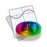Draw any 2D or 3D function almost instantly